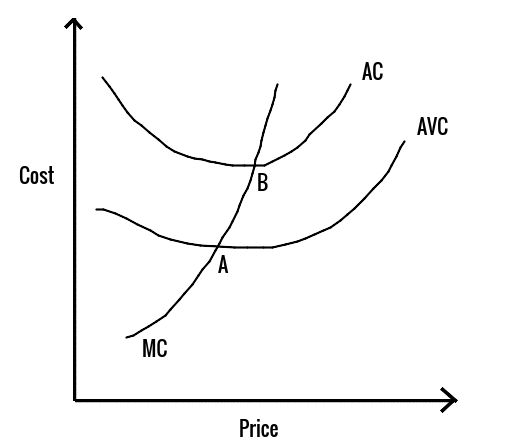# Shut-Down Analysis

The shutdown point refers to the minimum price or minimum unit of production required for the firm to keep continuing its operation. In other words, it is the minimum price or minimum unit of production at which the firm should exit the business by shutting down its operations.
• In the short run, the firm should be able to cover its variable cost through its sales revenue, in order to keep continuing its operation.
• Total Revenue from Operation - (Fixed Cost + Variable Cost) = Total Profit
• For the firm to continue its operation :
• Total Revenue from Operation = Total Variable Cost
• This is because the firm cannot avoid its fixed costs, but it should be able to cover the variable expenses.

#### Example:

A toy manufacturing firm produces 1000 units and sells them at a price of Rs 5 each. Then the Total Revenue from sales is (TR) is 5 × 1,000 = Rs 5,000. The Average Total Cost (AVC) is Rs 7,000 with a fixed cost (FC) of Rs 4000 and a variable cost (VC) of Rs 3,000 for all units.
Now, consider two scenarios :
a) Should the firm shut down its production immediately?
• If the firm shuts down its production immediately, it will incur zero variable cost. But it still has to pay Fixed Cost since this is unavoidable (e.g Rent of building has to be paid till the end of the lease). In this case, the firm earns nothing but still has to pay Rs 4,000 (this will be the loss in this scenario)
b) Should the firm continue with its production?
• If the firm continues with its production, it is earning Rs 5,000 and incurring a total cost of Rs 7,000. So the loss in this scenario is Rs 2,000.
• In Scenario (b), the firm experiences lesser loss, so it should continue with its production in the short term. But if the revenue from sales does not increase in the long term, it will have to shut down its operation.

#### Example:

A toy manufacturing firm produces 1000 units and sells them at a price of Rs 2 each. Then the Total Revenue from sales is (TR) is 2 × 1,000 = Rs 2,000. The Average Total Cost (AVC) is Rs 7,000 with a fixed cost (FC) of Rs 4000 and a variable cost (VC) of Rs 3,000 for all units.
Now consider two scenarios :
a) Should the firm shut down its production immediately?
• If the firm shuts down its production immediately, it will incur zero variable cost. But it still has to pay fixed cost since this is unavoidable (e.g rent of building has to be paid till the end of the lease). In this case, the firm earns nothing but still has to pay Rs 4,000 (this will be the loss in this scenario).
b) Should the firm continue with its production?
• If the firm continues with its production, it will be earning Rs 2,000 and incurring a total cost of Rs 7,000. So the loss in this scenario is Rs 5,000.
• In Scenario (a), the firm experiences lesser loss, so it should shut its production down immediately.
• Any firm will shut down its production when the marginal cost is less than average variable cost. Marginal Cost(MC) is the cost incurred in producing one more unit of a good.

### ShutDown Curve:AC: Average Cost
AVC: Average Variable Cost MC: Marginal Cost
• Point A is known as Shutdown Point because at this point Marginal Cost is the same as Average Variable Cost.
• Point B is known as Breakeven Point because at this point Marginal Cost is the same as Average Total Cost.

## Short Run and Long Run decision to operate or not

• Total Revenue from Sale ≥ Total Cost
• Short Term Decision: Stay in Market
• Long Term Decision: Stay in Market
• Total Revenue from Sales ≥ Total Variable Cost,&, Total Revenue from Sale ≤ Total Cost
• Short Term Decision: Stay in Market
• Long Term Decision: Shutdown Operation and Exit from Market
• Total Revenue from Sales < Total Variable Cost
• Short Term Decision: Shut down Production
• Long Term Decision: Exit from Market